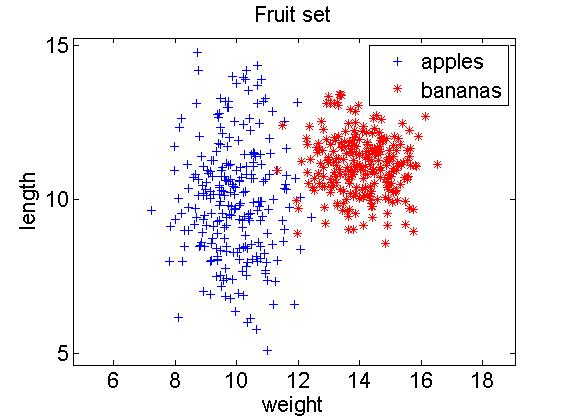### How to create a dataset from raw data?

(This FAQ is based on PRTools 5 or later, in which th `dataset` command was replaced by `prdataset`.)

This strongly depends on how the data is available. Important is that you separate data from labels. The Matlab command `load` may be used to read text data from disk. Before or after loading, the data should be organized as an` m x k `matrix of` m `objects given by` k `feature values. The labels should be supplied either numerical (doubles), as a character array or as a cell array of strings. The first dimension should always be` m`. After that

`>> a = prdataset(data,labels)`

creates the dataset . Use `setlablist` and `setprior` to add labels (class names) and prior probabilities if desired. Some examples:

```>> class_1 = gauss(250,[10 10],[1 0; 0 4]);    % collect data of class 1 >> class_2 = gauss(250,[14 11],[1 0; 0 1]);    % collect data of class 2 >> labels = genlab([250,250]);                 % generate labels (just 1's and 2's) >> a = prdataset([class_1;class_2],labels);    % create dataset >> a = setlablist(a,char('apples','bananas')); % change labels into real class names >> a = setfeatlab(a,char('weight','length'));  % give features a name >> a = setprior(a,[0.2,0.8]);                  % set priors >> a = setname(a,'Fruit set');                 % give the dataset a name >> a                                           % display summary of dataset Fruit set, 500 by 2 prdataset with 2 classes: [250  250] >> scatterd(a,'legend');                       % scatter plot with annotation >> axis equal >> struct(a)                                   % show structure describing the dataset ```

`        data: [500x2 double]`
`     lablist: {2x4 cell}`
`        nlab: [500x1 double]`
`     labtype: 'crisp'`
`     targets: []`
`     featlab: [2x6 char]`
`     featdom: {}`
`       prior: [0.2000 0.8000]`
`        cost: []`
`     objsize: 500`
`    featsize: 2`
`       ident: [1x1 struct]`
`     version: {[1x1 struct]  '16-Dec-2012 14:30:52'}`
`        name: 'Fruit set'`
`        user: []`